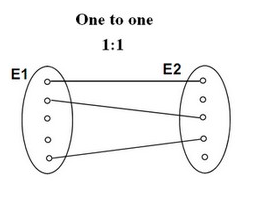# Set Theory - Bijection function (One-to-one relationship)

A Bijection is a mathematical / set function between the elements of two sets (ie binary function) where

• each element of one set is paired with exactly one element of the other set,
• each element of the other set is paired with exactly one element of the first set.
• there are no unpaired elements

This function is also known as:

## Example

• A bijection (Ref
• Not a bijection

## Properties

A function f: R → R is bijective if and only if its graph meets every horizontal and vertical line exactly once.

## Documentation / Reference

Discover MoreLanguage - Equality

The Equality operators model the Equivalence relation: equality is parallel to (for affine spaces) is in bijection with isomorphy The Equality operators are a subset of the comparison operator....Logical Data Modeling - Equivalence Relationship (Equality)

The equivalence relation is a relationship between two entities. The equivalence relation is also known as: is equal to. equality is parallel to (for affine spaces) is in bijection with - bijection...Logical Data Modeling - Primary Key (Id, Name)

A Primary Key is a key that identify indefinitely uniquely an element: entity or a in their local scope. It's a part of the fully qualified identifier. A real primary key is immutable as...Relational Data Modeling - One-to-One Relationship

A one-to-one relationship is a relational relationship that is equivalent to the set bijection relationship This relationship describe that one row from a relation point to exactly one row to the other...